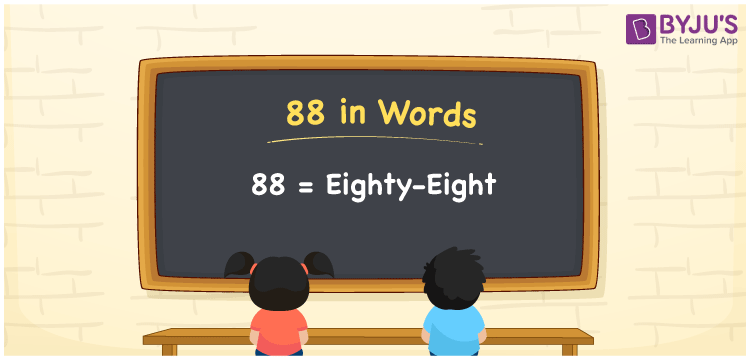# 88 in Words

88 in words is written as Eighty-Eight. If you have bought a geometry box worth Rs. 88, you can write “I have bought a geometry box worth Rupees Eighty-Eight”. 88 is a cardinal number since it shows a certain amount. With the help of a place value chart, we can write the number name of 88.

 88 in words Eighty-Eight Eighty-Eight in numerical form 88

## 88 in English Words

The English language is the most commonly used language in the field of Education. Numbers in words are expressed using the English alphabet. Hence, the English language is essential for students to learn how to write numbers in words. Thus, 88 in words is written as Eighty-Eight.## How to Write 88 in Words?

We can write the number name of 88 using the place value of a number. Hence, to write 88 in words, determine the place value of each digit of 88.

 Tens Ones 8 8

Thus, we can write the expanded form as:

8 × Ten + 8 × One

= 8 x 10 + 8 x 1

= 80 + 8

= 88

= Eighty-Eight

Therefore, 88 in words is written as Eighty-Eight.

Interesting way of writing 88 in words

8 = Eight

80 = Eighty

80 + 8 = 88

Eighty + Eight = Eighty-Eight

Thus, the word form of the number 88 is Eighty-Eight.

88 is a natural number. The successor of 88 is 89 and the predecessor of 88 is 87

• 88 in words – Eighty-Eight
• Is 88 an odd number? – No
• Is 88 an even number? – Yes
• Is 88 a perfect square number? – No
• Is 88 a perfect cube number? – No
• Is 88 a prime number? – No
• Is 88 a composite number? – Yes

## Frequently Asked Questions on 88 in Words

Q1

### How to write 88 in English?

We can write 88 in English as Eighty-Eight.
Q2

### What is the value of 88 minus 60?

88 minus 60 = 88 – 60 = 28 Therefore, the value of 88 minus 60 is 28.
Q3

### Is 88 an even number?

Yes, 88 is an even number.